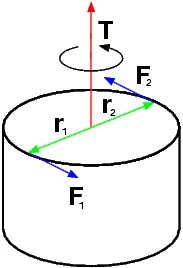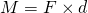Physics - Classical Mechanics - Force Couple System[Image 1]

Introduction

Hey it's a me again @drifter1! After a quite extensive pause, where I also finally got my Computer Science Degree, I'm back to rock! Today we continue with Physics and more specifically the branch "Classical Mechanics" to continue with the chapter of Equilibrium and Elasticity. In this article we will get into Force Couple Systems that are a great example of rigid body equilibrium. So, without further ado, let's dive straight into it!

Force Couple System

A couple consists of two parallel forces. Those forces are equal in magnitude but opposite. The resultant force is zero, because the forces in a couple do not share a line of action. A couple produces only rotation and not translational movement. If the forces were unequal an unbalanced force would be produces, causing a translation of the system.

The moment of a couple is equal to the product of the magnitude of one of the forces and the perpendicular distance between their lines of action:The magnitude of the moment of a couple is equal for all points in the plane of the couple. The magnitude is independent of the reference point.

A couple cannot be put in equilibrium by a single force, but only another couple of equal magnitude and opposite direction anywhere in the same plane or in a parallel plane. Combining couples together we form a couple with a moment equal to their algebraic sum.

Example from 

The stem of a valve has two hand wheels. A small wheel of 30cm diameter that is used to spin the valve quickly as it is opened and closed, and a larger 80 cm wheel that may be used to free a stuck valve or seat the valve tighly when it is fully closed. Lets suppose a operator that can apply a force of 150N on each side of the large wheel. This creates a force couple. What is the moment imposed on the valve stem?

Lets use scalars to solve the problem. The perpendicular distance between the two forces is equal to the diameter of the larger wheel and so 80 cm. Lets make the origin of the axes equal to the point one of the two forces is applied to. Lets also suppose an x axis that is perpendicular to both forces and passing through the point they are applied to and an y axis parallel to the forces.

That way the moment is calculated as follows:RESOURCES:

Images

1.Previous articles of the series

Equilibrium and Elasticity

• Rigid Body Equilibrium -> Equilibrium Conditions of Rigid Bodies, Center of Gravity, Solving Equilibrium Problems

Final words | Next up

And this is actually it for today's post!

Next time we will continue with Tensile Stresses and Deformations...

See ya!Keep on drifting!

H2
H3
H4
3 columns
2 columns
1 column